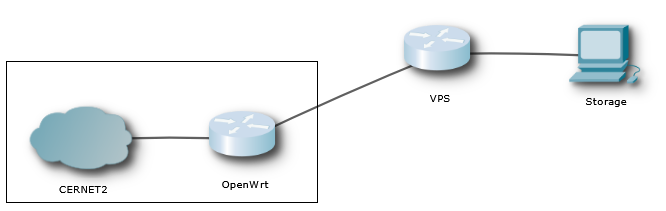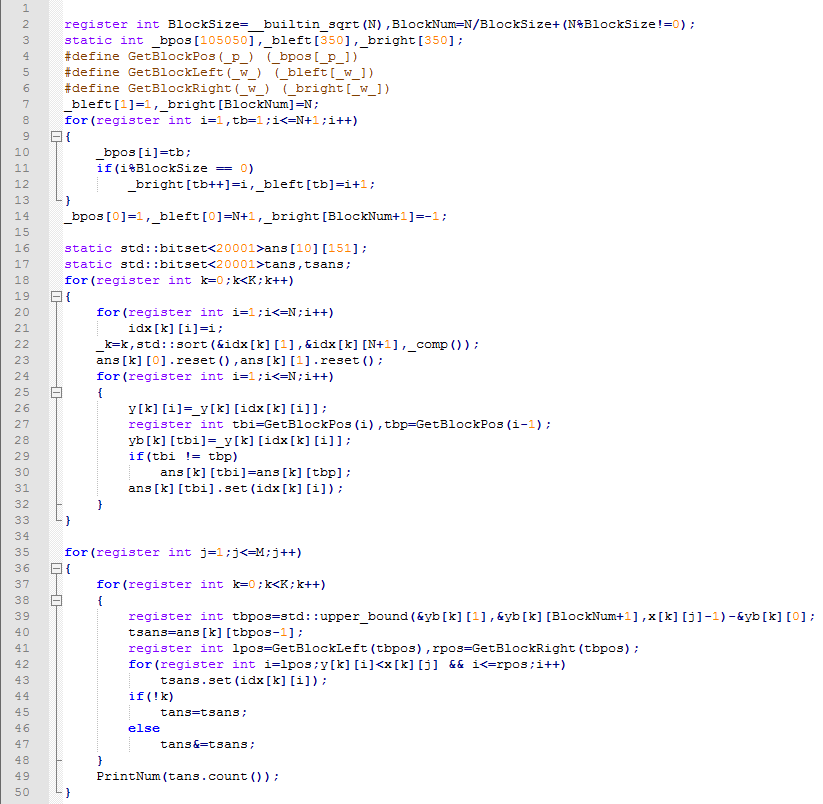## OpenWrt 打通 IPv6 校园网…

…

…

…

# [研学] 质因数分解及素性判定

• ### 【1.1】记号与规定

1. 记 $$\displaystyle\mathbb{R}$$ 表示实数集，$$\displaystyle\mathbb{N}$$ 表示自然数集，$$P$$ 表示素数集。
2. 勒让德符号 $$\displaystyle\left( {\frac{n}{p}} \right)$$。设 $$\displaystyle p \in P,n \in \mathbb{N}$$。

• ### 【1.2】素数的一些性质

• $$P$$ 是无限集。
• 对于任意大于 $$1$$ 的自然数，它要么是个素数，要么可以分解为若干素数之积，并且在忽略顺序的情况下，这样的分解是唯一的。
• 小于 $$n$$ 的质数大约有 $$\ln n$$ 个。
• 一个合数 $$n$$ 最小的素因数因数一定小于 $$\sqrt n$$。
• 费马小定理：设 $$p$$ 是大于 $$2$$ 的素数，则对于任意正整数 $$n$$ 均有 $$\displaystyle \begin{array}{*{20}{c}}{{n^{p – 1}} \equiv 1}&{(\bmod p)}\end{array}$$。
• Mertens’ second theorem

# [研学] 偏序问题的研究

• ### 高维偏序…